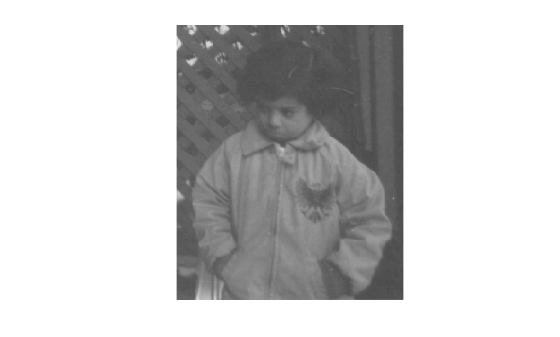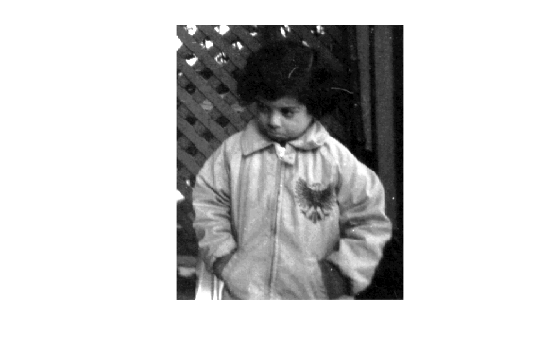Documentation

stretchlim

Find limits to contrast stretch image

Description

example

lowhigh = stretchlim(I) computes the lower and upper limits that can be used for contrast stretching grayscale or RGB image I. The limits are returned in lowhigh. By default, the limits specify the bottom 1% and the top 1% of all pixel values.

You optionally can calculate the limits using a GPU (requires Parallel Computing Toolbox™).

lowhigh = stretchlim(I,Tol) specifies the fraction, Tol, of the image to saturate at low and high pixel values.

Examples

collapse all

Read grayscale image into the workspace and display it.

figure
imshow(I)Adjust the contrast in the image using stretchlim to set the limits, and display the result. The example uses the default limits [0.01 0.99], saturating the upper 1% and the lower 1%.

figure
imshow(J)Input Arguments

collapse all

Image to be contrast stretched, specified as a 2-D grayscale image or 2-D RGB image.

Example: I = imread('pout.tif');

Example: I = imread('peppers.png');

Data Types: single | double | int16 | uint8 | uint16

Fraction of the image to saturate, specified as a numeric scalar or 2-element vector [Low_Fract High_Fract] in the range [0 1].

ValueDescription
ScalarIf Tol is a scalar, then Low_Fract = Tol, and High_Fract = 1 - Low_Fract, which saturates equal fractions at low and high pixel values.
0If Tol = 0, then lowhigh = [min(I(:)); max(I(:))].
DefaultIf you omit the Tol argument, then [Low_Fract High_Fract] defaults to [0.01 0.99], saturating 2%.
Too bigIf Tol is too big, such that no pixels would be left after saturating low and high pixel values, then stretchlim returns [0 1].

Example: lohi = stretchlim(I,[.02 .80]);

Data Types: single | double | int8 | int16 | int32 | int64 | uint8 | uint16 | uint32 | uint64

Output Arguments

collapse all

Lower and upper limits for contrast stretching, returned as one of the following.

• A 2-element numeric vector when I is a grayscale image.

• A 2-by-3 numeric matrix when I is an RGB image. The columns indicate the lower and upper limit for each of the three color channels.

Data Types: double

Tips

• Use the imadjust function to adjust the contrast of image I using the limits, lowhigh.